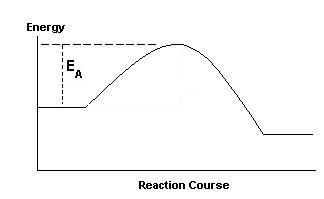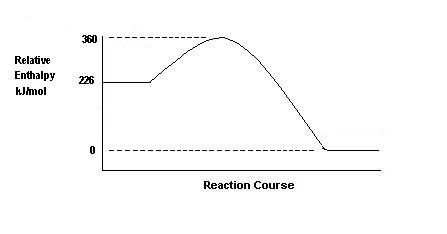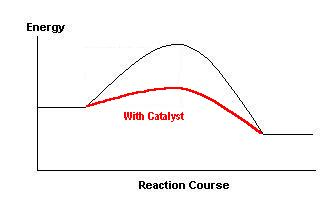Kinetics

For on-line tutorial help with kinetics, there are four modules available in the Kinetics section of chemcal.

Shortcut to Questions

Q: 1 2 3 4 5 6 7 8

1

(a) What is the meaning of EA on the diagram?(b) Indicate on the diagram the effect of the presence of a catalyst.2

Given below is a potential energy diagram for the reaction

CO(g) + NO2(g) → CO2(g) + NO(g)(a) What is the activation energy for the reaction?
(b) What is the activation energy for the reverse reaction?
(c) What is the value of ΔH for the reaction?
(d) Explain briefly the effect of an increase in temperature on the rate of the forward reaction.3

For the reaction

2A + B + C → 3D + E

the rate of formation of E is found to double if [A] is doubled, keeping [B] and [C] constant, and is found to double if [B] is doubled keeping [A] and [C] constant. The rate is unaffected by changes in [C]. Write the rate law for the reaction.4

For the reaction 2NO(g) + O2(g) → 2NO2(g) state the relationship between the rate of consumption of NO, the rate of consumption of O2, and the rate of production of NO2.5

The reaction between nitrite ion and iodide ion in acidic solution can be represented by the equation:

2NO2- + 2I- + 4H+ → I2 + 2NO + 2H2O

The experimental rate law for this reaction is
R = k[NO2-][I-][H+]2
By what factor, if any, would the rate of reaction be altered if-

(a) [H+] and [I-] were kept constant but [NO2-] were doubled?
(b) [I-] and [NO2-] were kept constant but [H+] were doubled?
(c) [I-] and [NO2-] were kept constant but [H+] were halved?
(d) All concentrations were doubled?6

The rate of the reaction   H3O+ + NH3  →  NH4+ + H2O  was studied in 1960 and the rate expression shown to be -

R = k[H3O+][NH3]

where k = 4.3 x 1010 litre mole-1 second-1 at 298 K.  Calculate the rate of this acid-base reaction the instant 0.10 M ammonia (400 cm3) is mixed with 0.050 M nitric acid (200 cm3).7 Benzene diazonium chloride is found to undergo hydrolysis as follows:

C6H5N2Cl  +  2H2O  →  C6H5OH  +  H3O+  +  N2  +  Cl-

At 298 K, the initial rate of hydrolysis was found to increase with the initial concentration of benzene diazonium chloride as follows:

 Initial concentration (mole litre-1) 0.0200 0.0400 Initial rate (mole litre-1 second-1) 8.70 x 10-7 1.74 x 10-6

(a) Use these results to determine the value of the reaction order, n, in the rate equation:

Rate of formation of phenol = rate constant x [C6H5N2Cl]n

(b) Hence calculate the rate constant, k, in the rate equation above.

(c) The rate of the reaction can be expressed as the derivative

d[C6H5OH] / dt

Choose an alternative derivative expression for the rate, and give the relationship between the two differentials.8

Dinitrogen pentoxide decomposes in the solvent carbon tetrachloride according to the following stoichiometric equation

2N2O5  →   4NO2  +  O2

The following data concerns this decomposition at 303 K.

 Reactant concentration  (M) Decomposition rate: -d [N2O5] / dt (mole litre-1 hour-1) 0.170 0.050 0.340 0.10 0.680 0.20

(a) Write the rate equation for the decomposition.

(b) Give the reaction order for the decomposition.

(c) Calculate the rate constant for the decomposition reaction at 303 K.1

(a) EA is the activation energy for the forward reaction. This is the minimum energy that the colliding reactant molecules must possess before their collisions can lead to the formation of product molecules.

(b) The effect of the catalyst is to lower the height of the "hump". By offering a new route the presence of the catalyst lowers EA (for both forward and backward reactions).2

(a) From the graph, EA for the forward reaction = (360 - 226)

= 134 kilojoule mole-1

(b) From the graph, EA for the reverse reaction = (360 - 0)

= 360 kilojoule mole-1

(c) The enthalpy change, ΔH = (0 - 226) = -226 kilojoule mole-1

(d) At higher temperatures the proportion of molecules with the necessary activation energy is increased. Rate of forward (and backward) reaction is thus increased.3

Note that unlike equilibrium constants, a rate law expression cannot be deduced from the stoichiometric equation. Instead it requires a series of separate experiments to deduce in turn the effect that the concentration of each reactant has on the overall rate of the reaction. In a series of experiments, only one reactant's concentration is altered in each experiment while the concentrations of all the other reactants are held unchanged. By these means, the effect of changing the concentration of each reactant in turn on the rate of the overall reaction can be determined.
In the first experiment, doubling the concentration of A (written as [A]) alone doubles the rate of formation of E, so the reaction rate is directly proportional to [A].
i.e. rate = constant x [A]1.
In the second experiment, keeping [A] and [C] fixed while doubling [B] alone causes the rate of formation of E to double, so the reaction rate is directly proportional to [B] as well.
i.e. rate= constant x [B]1.
In the third experiment to establish the effect of changing the concentration of C alone, the rate of the reaction is not altered so [C] does not appear in the rate law for the reaction,
or rate = constant x [C]0.
Combining these results,
rate = k[A]1[B]1[C]0 , or rate = k[A][B]4

In this question, the rate law is not requested and in fact the data needed to deduce it is not given. Here the simple stoichiometric relationship between the various reactants and products is all that is required to establish their relative rates of consumption or formation. In general terms, suppose that 1 molecule of a reactant A uses 2 molecules of another reactant B to form 1 molecule of product C, then the rate of consumption of B is twice that of A and that the rate of formation of C is identical to the rate of consumption of A.

In this question, 2 molecules of NO reacts with 1 molecule of O2 so the rate of consumption of NO must be twice that of O2 while each molecule of NO forms 1 molecule of NO2, so the rate of consumption of NO is the same as the rate of formation of NO2.

By convention, the consumption of a reactant is shown by attaching a negative sign to its rate while the formation of a product is indicated by a + sign associated with its rate.

In words, the rate of consumption of NO equals the rate of formation of NO2, and the rate of disappearance of O2 is one-half this rate.

Or, using the calculus notation of d[A]/dt for the rate of change of concentration of any species A,

2 x -d[NO]/dt = -d[O2]/dt = + 2 x d[NO2]/dt5 (a) If only the [NO2-] is altered and is doubled, then from the rate law, this would cause the rate to double as rate [NO2-]1.

(b) If only the [H+] is altered and is doubled, then from the rate law, this would cause the rate to increase by a factor of 4 as rate [H+]2.

(c) If only the [H+] is altered and is halved, then from the rate law, this would cause the rate to decrease to ¼ of the original rate as rate ∝ [H+]2, i.e. ( ½. )2 = ¼.

(d)   If all concentrations were doubled, then the doubling of [NO2-] would double the rate and doubling the [I-] would double the rate again (now increased by a factor of 4) and doubling the [H+] would increase the rate by a further factor of 4 to give a total increase in rate of 16 times the original rate.6

The rate law is rate = 4.3 x 1010 [H3O+][NH3].

Calculate the instantaneous [H3O+] and [NH3] which have been diluted upon mixing as follows:

Total volume.= 600 mL.

For dilutions, the expression M1V1 = M2V2 is valid.

for the diluted [H3O+], 0.050 x 200 = M2 x 600

so [H3O+] = 1.67 x 10-2 M

and for the diluted [NH3], 0.10 x 400 = M2 x 600

so [NH3] = 6.66 x 10-2 M

Substituting these values for [H3O+] and [NH3] in the rate law

Rate = 4.3 x 1010 [H3O+][NH3]

= 4.3 x 1010 (1.67 x 10-2)(6.66 x 10-2)

= 4.8 moles litre-1 second-1  (4.8 M s-1)7 (a)   By inspection of the data, doubling the [C6H5N2Cl] causes the rate to increase from 8.70 x 10-7 M s-1 to 1.74 x 10-6 M s-1, i.e. to double.

rate is directly proportional to the [C6H5N2Cl], so n = 1.

(b)   Substitute either set of data in the rate equation.

8.70 x 10-7 = k x 0.0200

k = 4.35 x 10-5 s-1.

(c)   See answer to Question 4 above for a discussion of the background to this part.

From the stoichiometry of the equation, each mole of C6H5OH produced corresponds to the consumption of one mole of C6H5N2Cl. Hence an alternative differential expression for the rate would be

-d [C6H5N2Cl] /dt      (The - sign indicates that species is being consumed)

The relation between them is

d [C6H5OH] /dt =  -d [C6H5N2Cl] /dt8 (a) By inspection of the data, doubling the concentration of N2O5 causes the rate to double, so the rate law is rate = k[N2O5]1.

(b)   As n = 1, reaction order = 1.

(c) To evaluate k, substitute any of the sets of data.

0.10 = k x 0.340

k = 2.9 x 10-1 hr-1.